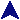Exam I Study GuideCSc 231 Study GuidesHere are some things which would be good to know for the first exam. You should also look at the practice problems.
1. Basic Data Structures.
1. Know how to create strings using `"`, `'`, `q`, and `qq`.
2. Know how variables are interpolated into strings.
3. Know how `\$`, `@` and `%` identify scalars, arrays, and hashes respectively.
4. Know how to subscript arrays and hashes.
5. Know how to find the maximum subscript of an array.
6. Know how to use the array operations such as `shift`.
7. Know how list assignments work, including mixing lists and arrays.
8. Know how to get the keys out of a hash.
9. Know what `@ARGV` and `%ENV` are.
10. Remember that the C/C++/Java `++` and `--` operators, in prefix and postfix forms, are still here to haunt you.
11. Know what `\$_` is, and what is used for.
2. Control Structures
1. Understand the `while`, `for` and `foreach` loops.
2. Know the `last`, `next`, and `redo` statements, and how they differ.
3. Understand how to use `shift` with `while`.
4. Know how to use `die`.
3. I/O.
1. Know how to read and write standard input and output.
2. Know what `chomp` is for.
3. Know how to open files with `open`, for reading or for writing.
4. Know how to test files with the `-x` operators (`-e`, `-x`, etc.)
5. Know how to print using a file handle.
6. Know how to read using a file handle: The `<FI>` notation.
4. Functions.
1. Know how to create a function (perl `sub`).
2. Know how to create local variables with `my` (and that other references are global).
3. Know what `@_` is.
4. Know how parameters are passed, and how a `sub` can use them.
5. Know that parameter lists are flattened into a single list before being sent, merging everything into one array.
5. Be able to understand and create patterns. Here are some particular things to concentrate on.
1. Know what the `=~` and `!~` operators do.
2. Know how to create and use pattern matches and pattern replacements.
3. Know how to use at least the following pattern operators: `*`, `+`, `^`, `\$`, `{}`, `[]`, `.` and `\s`.
4. Know how to `^` behaves in and out of `[]`.
5. Know how to use parentheses to capture a portion of a match into `\$`n variables.
6. Know that patterns interpolate variables.
6. Know what to do with the `system` call.
7. References, packages, and modules.
1. Know how to create a reference to an existing variable, and how to use a reference.
2. Know how to pass references as parameters, and how to use them.
3. Know how to create a package, and how to create a module.
4. Know what a blessed reference is, and how to create one.
5. Know how a method call of the form `\$r->f(x)`, for a blessed reference `\$r`, is translated.
6. Know how to create objects in perl.